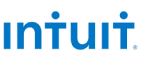New update is available. Click here to update.
Topics

# Distance to a Cycle in Undirected Graph

Moderate0/80
1 upvote## Problem statement

You are given a graph with ‘N’ nodes and exactly one cycle. You are given a 2-d array ‘EDGES’, where ‘Edges[i]’=[‘node0’, ’node1’], denotes an undirected edge from node0 to node1. The nodes are numbered from 0 to ‘N’-1, where ‘N’ is the number of nodes in the graph.

The distance between two nodes is defined as the number of edges between them.

You must return an array ‘ANSWER’ of size ‘N’, where ‘ANSWER[i]’ is the shortest distance between node ‘i’ and any node present in the cycle.

Note :

``````The graph is connected.
There is, at most, one edge between any pair of nodes.
There is exactly one cycle in the graph.
``````
Example:
``````Input: N=7, edges=[[1, 2], [2, 3], [3, 4], [4, 1], [0, 1], [5, 2], [6, 5]]

Output: [1, 0, 0, 0, 0, 1, 2]

Explanation: The Nodes 1, 2, 3, and 4 form a cycle.
The distance between nodes 0 to 1 is 1.
The distance between nodes 1 to 1 is 0.
The distance between nodes 2 to 2 is 0.
The distance between nodes 3 to 3 is 0.
The distance between nodes 4 to 4 is 0.
The distance between nodes 5 to 2 is 1.
The distance between nodes 6 to 2 is 2.
``````
Detailed explanation ( Input/output format, Notes, Images )
Constraints :
``````1 <= 'T' <= 10
3 <= 'N' <= 10^5
0 <= ‘node0’, ‘node1’ <=’N’-1
‘node0’ != ’node1’

Time Limit: 1 sec
``````
Sample Input 1 :
``````2
7
0 1
1 2
1 3
2 4
2 5
3 4
5 6
3
0 1
1 2
0 2
``````
Sample Output 1 :
``````1 0 0 0 0 1 2
0 0 0
``````
Explanation Of Sample Input 1 :
``````For test case 1:

Input: N=7, edges=[[1, 2], [2, 3], [3, 4], [4, 1], [0, 1], [5, 2], [6, 5]]

Output: [1, 0, 0, 0, 0, 1, 2]

Explanation: The Nodes 1, 2, 3, and 4 form a cycle.
The distance between nodes 0 to 1 is 1.
The distance between nodes 1 to 1 is 0.
The distance between nodes 2 to 2 is 0.
The distance between nodes 3 to 3 is 0.
The distance between nodes 4 to 4 is 0.
The distance between nodes 5 to 2 is 1.
The distance between nodes 6 to 2 is 2.

For test case 2:

Input: N=3, edges=[[0, 1], [1, 2], [0, 2]]

Output: [0, 0, 0]

Explanation: The Nodes 0, 1, and 2 form a cycle.
The distance between nodes 0 to 0 is 0.
The distance between nodes 1 to 1 is 0.
The distance between nodes 2 to 2 is 0.
``````
Sample Input 2 :
``````2
9
0 1
1 2
0 2
2 6
6 7
6 8
1 3
3 4
3 5
4
0 1
1 2
2 3
3 1
``````
Sample Output 2 :
``````0 0 0 1 2 2 1 2 2
1 0 0 0
``````Console